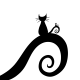This Scripting Algebra (SA) activity looks at quadrants, mouse location, and interactive art p5.js.

This activity assumes that the skills from SA1-SA8 were introduced (if-else, logical operators, random(), text(), rect(), variables, loop, pow, for, functions, translate, modulo, etc.). This activity encourages learners to create quadrant art and leverage previous concepts and code.

Save work often and have fun (or just start over if you break it).

• Analyze the code below.
• What symmetries do you see?
• Using the conditional statements for each quadrant, create your own work of art.
• Use shapes and concepts from previous tasks in this project
• Example Code at p5.js.

See the Pen QuadrantArt by Sophia (@fractalkitty) on CodePen.

• This example is similar to the odd code above as an example for an individual’s project.
• Example Code at p5.js.

See the Pen QuadrantArt2 by Sophia (@fractalkitty) on CodePen.

Example Concepts: cartesian coordinates, quadrants, interactive code, variables, coordinate planes, ordered pairs, conditions, loops, random numbers, functions, etc..

## Like what you see?Become a Patron!